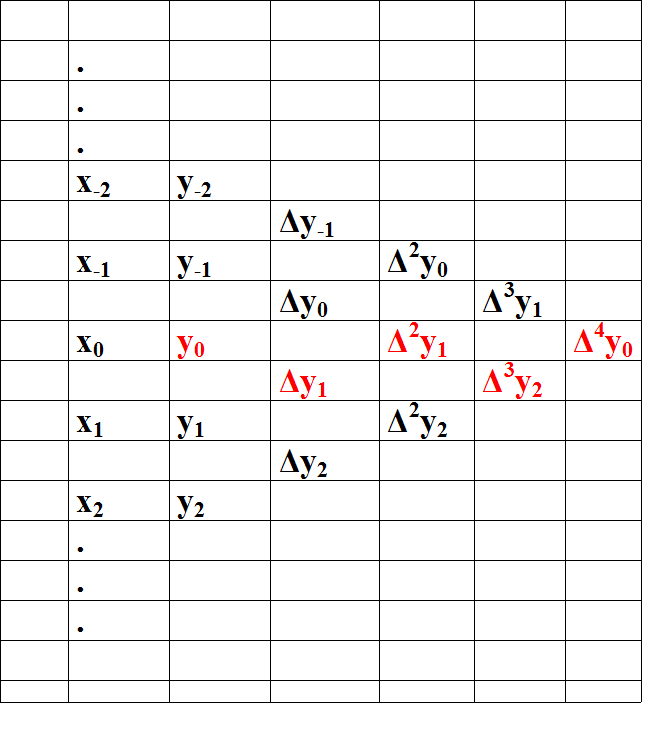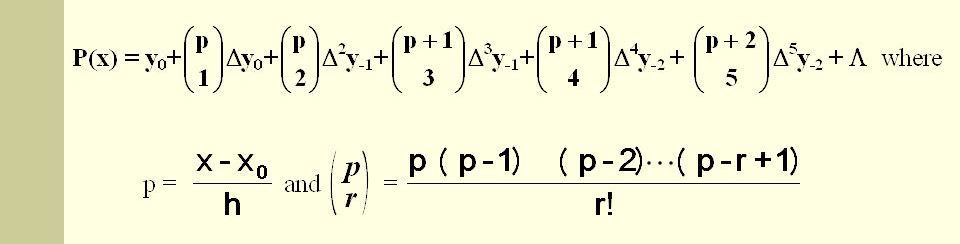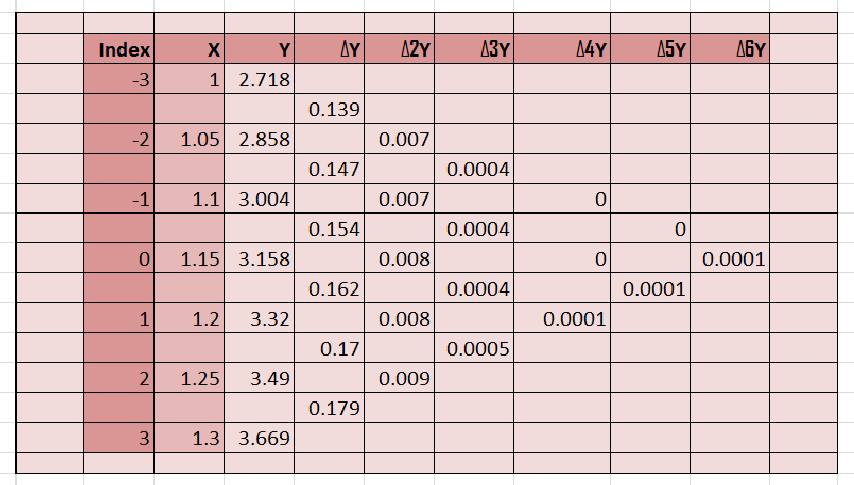Related Articles

# Gauss’s Forward Interpolation

• Last Updated : 17 Jun, 2021

Interpolation refers to the process of creating new data points given within the given set of data. The below code computes the desired data point within the given range of discrete data sets using the formula given by Gauss and this method known as Gauss’s Forward Method.

Attention geek! Strengthen your foundations with the Python Programming Foundation Course and learn the basics.

To begin with, your interview preparations Enhance your Data Structures concepts with the Python DS Course. And to begin with your Machine Learning Journey, join the Machine Learning - Basic Level Course

Gauss’s Forward Method:

The gaussian interpolation comes under the Central Difference Interpolation Formulae. Suppose we are given the following value of y=f(x) for a set values of x:
X: x0 x1 x2 ………. xn
Y: y0 y1 y2 ………… yn
The differences y1 – y0, y2 – y1, y3 – y2, ……, yn – yn–1 when denoted by Δy0, Δy1, Δy2, ……, Δyn–1 are respectively, called the first forward differences. Thus the first forward differences are :
Δy0 = y1 – y0
and in the same way we can calculate higher order differences.And after the creating table we calculate the value on the basis of following formula:Now, Let’s take an example and solve it for better understanding.
Problem:
From the following table, find the value of e1.17 using Gauss’s Forward formula.

Solution:
We have

yp = y0 + pΔy0 + (p(p-1)/2!).Δy20 + ((p+1)p(p-1)/3!).Δy30 + …
where p = (x1.17 – x1.15) / h
and h = x1 – x0 = 0.05
so, p = 0.04
Now, we need to calculate Δy0, Δy20, Δy30 … etc.Put the required values in the formula-
yx = 1.17 = 3.158 + (2/5)(0.162) + (2/5)(2/5 – 1)/2.(0.008) …
yx = 1.17 = 3.2246
Code : Python code for implementing Gauss’s Forward Formula

## Python3

 `# Python3 code for Gauss's Forward Formula``# importing library``import` `numpy as np` `# function for calculating coefficient of Y``def` `p_cal(p, n):` `    ``temp ``=` `p;``    ``for` `i ``in` `range``(``1``, n):``         ``if``(i``%``2``=``=``1``):``             ``temp ``*` `(p ``-` `i)``         ``else``:``             ``temp ``*` `(p ``+` `i)``    ``return` `temp;``# function for factorial``def` `fact(n):``    ``f ``=` `1``    ``for` `i ``in` `range``(``2``, n ``+` `1``):``        ``f ``*``=` `i``    ``return` `f` `# storing available data``n ``=` `7``;``x ``=` `[ ``1``, ``1.05``, ``1.10``, ``1.15``, ``1.20``, ``1.25``, ``1.30` `];` `y ``=` `[[``0` `for` `i ``in` `range``(n)]``        ``for` `j ``in` `range``(n)];``y[``0``][``0``] ``=` `2.7183``;``y[``1``][``0``] ``=` `2.8577``;``y[``2``][``0``] ``=` `3.0042``;``y[``3``][``0``] ``=` `3.1582``; ``y[``4``][``0``] ``=` `3.3201``;``y[``5``][``0``] ``=` `3.4903``;``y[``6``][``0``] ``=` `3.6693``;` `# Generating Gauss's triangle``for` `i ``in` `range``(``1``, n):``    ``for` `j ``in` `range``(n ``-` `i):``        ``y[j][i] ``=` `np.``round``((y[j ``+` `1``][i ``-` `1``] ``-` `y[j][i ``-` `1``]),``4``);` `# Printing the Triangle``for` `i ``in` `range``(n):``    ``print``(x[i], end ``=` `"\t"``);``    ``for` `j ``in` `range``(n ``-` `i):``        ``print``(y[i][j], end ``=` `"\t"``);``    ``print``("");` `# Value of Y need to predict on``value ``=` `1.17``;` `# implementing Formula``sum` `=` `y[``int``(n``/``2``)][``0``];``p ``=` `(value ``-` `x[``int``(n``/``2``)]) ``/` `(x[``1``] ``-` `x[``0``])` `for` `i ``in` `range``(``1``,n):``    ``# print(y[int((n-i)/2)][i])``    ``sum` `=` `sum` `+` `(p_cal(p, i) ``*` `y[``int``((n``-``i)``/``2``)][i]) ``/` `fact(i)` `print``(``"\nValue at"``, value,``    ``"is"``, ``round``(``sum``, ``4``));`

Output :

```1       2.7183  0.1394  0.0071  0.0004  0.0     0.0     0.0001
1.05    2.8577  0.1465  0.0075  0.0004  0.0     0.0001
1.1     3.0042  0.154   0.0079  0.0004  0.0001
1.15    3.1582  0.1619  0.0083  0.0005
1.2     3.3201  0.1702  0.0088
1.25    3.4903  0.179
1.3     3.6693

Value at 1.17 is 3.2246```

My Personal Notes arrow_drop_up